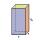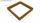# The tank

The tank has 1320 liters of water. The tank has the shape of a prism, its base is an rectangle with sides a = 0,6 m and b = 1,5 m. How high does the water level reach in the tank?

Result

h =  1.467 m

#### Solution:Leave us a comment of example and its solution (i.e. if it is still somewhat unclear...):

Showing 0 comments:Be the first to comment!#### To solve this example are needed these knowledge from mathematics:

Do you know the volume and unit volume, and want to convert volume units?

## Next similar examples:

1. Cylindrical tank 2If a cylindrical tank with volume is used 12320cm raised to the power of 3 and base 28cm is used to store water. How many liters of water can it hold?
2. Water reservoirThe water tank has a cuboid with edges a= 1 m, b=2 m , c = 1 m. Calculate how many centimeters of water level falls, if we fill fifteen 12 liters cans.
3. RainfallA rectangular garden of 25m in length and width 20m in width fall 4mm of water. Express by a fraction in basic form what part of the 60-hectolitre tank we would fill with this water.
4. Fire tankHow deep is the fire tank with the dimensions of the bottom 7m and 12m, when filled with 420 m3 of water?
5. HectolitersHow many hectoliters of water fits into cuboid tank with dimensions of a = 3.5 m b = 2.5 m c = 1.4 m?
6. RainfallOn Thursday, fell 1 cm rainfall. How many liters of water fell to rectangular garden with dimensions of 22 m x 35 m?
7. AquariumCan 30 liters of water fit in a cuboid aquarium with dimensions a = 3dm b = 6dm c = 5dm?
8. AquariumAquarium is rectangular box with square base containing 76 liters of water. Length of base edge is 42 cm. To what height the water level goes?
9. Pool in litresPool has a width of 3.5 m length of 6 m and a height 1.60 meters. Calculate pool volume in liters.
10. The shopThe shop has 3 hectoliters of water. How many liter bottles is it?
11. Children's poolChildren's pool at the swimming pool is 10m long, 5m wide and 50cm deep. Calculate: (a) how many m2 of tiles are needed for lining the perimeter walls of the pool? (b) how many hectoliters of water will fit into the pool?
12. Swimming pool 4The pool shaped cuboid measuring 12.5 m × 640 cm at the bottom is 960hl water. To what height in meters reaches the water level?
13. Concrete pillarHow many m³ of concrete is needed for the construction of the pillar shape of a regular tetrahedral prism, when a = 60 cm and the height of the pillar is 2 meters?
14. Building baseExcavation for the building base is 350x600x26000. Calculate its volume in m3.
15. Rectangular prismIf i have a rectangular prism with a length of 1,000 cm, width of 30 cm and a height of 50 cm, what is the volume?
16. Milk packageMilk is sold in a box with dimensions of 9.5 cm; 16.5 cm and 6.5 cm. Determine the maximum amount of milk that can fit into a box. Coating thickness is negligible.
17. Two cuboidsFind the volume of cuboidal box whose one edge is: a) 1.4m and b) 2.1dm